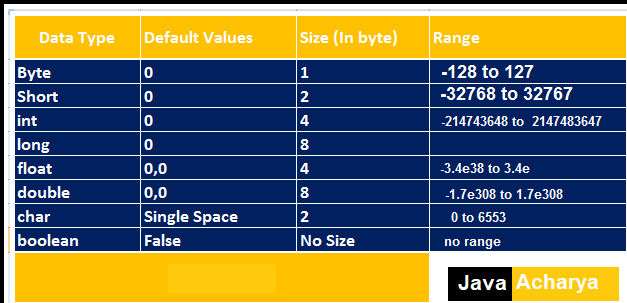Data Type in Java With Example

Data Types in java

Data type : In java every variable has a type , every expression has a type and all the type strictly define more over assignment should be checked by the compiler by the type compatibility hence java language considered as strongly typed language .
• To represent type of variable use data types
• To representing how much memory is allocated for a variable use data types
• To specifies range value of variable use data typesData types in Java
Types Of Data Types
There are two type of data types in java which are listed below .
1) Primitive data types - There are 8 primitive data types in java ( byte , Short , int , long , float , double , char , boolean )
2) Non Primitive data types - The non-primitive data type include (Classes , interface , Arrays)

Primitive Data Types
In java programming primitive data type are used to represent the types of variable and expression.
In Java Primitive data type divided into 8 part ..
1)byte data types
2) short data types
3) int data types
4) long data types
5) float data types
6) double data types
7) char data types
8) boolean data typesPrimitive Data Types in java
Byte data types
In java Language The byte data type is an 8-bit signed two’s complement integer. The range value of byte data type lies between (-128 to 127) where -128 is minimum value and 127 is maximum values. Default value of byte data type is zero (0).
Syntax - byte a=10
Size - 1 byte (8 bits)
Value- (-128 to 127)
Default value - (0)

Short Data Types

Short data types is most rarely used data type in java.The short data type is a 16-bit signed two’s complement integer. The range value of short data type lies between (-32 ,678 to 32 ,767 (inclusive)). where -32 ,678 is minimum value and 32 ,767 is maximum values. Default value of short data type is zero (0).
Syntax - short s=1000
Size - 2 byte (16 bits)
Value- (-32 ,678 to 32 ,767 (inclusive))
Default value - (0)

Int Data Type
This is most commonly used data type in java.The int data type is a 32-bit signed two's complement integer.The range value of int data type lies between -2,147,483,648 (-2^31) to 2,147,483,647 (2^31 -1) (inclusive)). where -2,147,483,648 is minimum value and 2,147,483,647 is maximum values. Default value of int data type is zero (0).
Syntax - int s=1000
Size - 4 byte (32 bits)
Value- (-2,147,483,648 (-2^31) to 2,147,483,647 (2^31 -1) (inclusive))
Default value - (0)

Long Data Type
Whenever int is not enough to holding the values then we should go for long data types. The range value of long data type lies between -9,223,372,036,854,775,808(-2^63) to 9,223,372,036,854,775,807(2^63 -1)(inclusive). where  -9,223,372,036,854,775,808 is minimum value and 9,223,372,036,854,775,807 is maximum values. Default value of long data type is zero (0).
Syntax - long s=100000L
Size - 8 byte (64 bits)
Value- (-9,223,372,036,854,775,808(-2^63) to 9,223,372,036,854,775,807(2^63 -1)(inclusive).)
Default value - (0)

Float Data Type
The value range for float data type is unlimited.
Syntax - float f1 =123.4F
Size - 4 byte (32 bits)
Value- Up to seven(7) decimal digits
Default value - (0.0F)

Double Data Type
The double data type is a double-precision 64-bit IEEE 754 floating point.The value range for double data type is unlimited. Default value of double data type is (0.0d)
Syntax - double d1 =12.3
Size - 8 byte (64 bits)
Value- Up to sixteen(16)decimal  digits
Default value - (0.0d)

Char Data Type
The char data type is a single 16-bit Unicode character.The value range for char data type is lies between '\u0000' (or 0) to '\uffff' (or 65,535 inclusive). Default value of char data type is ('\u0000').
Syntax - char ch='A'
Size - 2 byte (16 bits)
Value'\u0000' (or 0) to '\uffff' (or 65,535 inclusive)
Default value - ('\u0000')

Boolean Data Type
Boolean data type is used to represent the two possible value TRUE and FALSE.it specifies one bit of information, but its "size" can't be defined precisely.
Default value of boolean data type is false.
Syntax - boolean d=false
Size - virtual machine dependent
Value- true ,false
Default value - (false)

Declaration of data type in java
Syntax - data type  Name of variable = Value ;
Example - int a =10;
int- Data type
a - Variable name
= - Assignment
10- Constant Value
; - Statement terminator .

Hope !!! The Above Lesson of java Data types helpful For you ...

Technology Team,

UPCS
UP Consultancy Services

Tags : data type in java , java data types , types of data types , Primitive data type in java , reference data type in java .